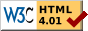#Boost C++ Libraries

...one of the most highly regarded and expertly designed C++ library projects in the world.

This is the documentation for an old version of boost. Click here for the latest Boost documentation.# Simple Segregated Storage

## Introduction

simple_segregated_storage.hpp provides a template class simple_segregated_storage that controls access to a free list of memory chunks. Please note that this is a very simple class, with preconditions on almost all its functions. It is intended to be the fastest and smallest possible quick memory allocator — e.g., something to use in embedded systems. This class delegates many difficult preconditions to the user (i.e., alignment issues). For more general usage, see the other pool interfaces.

## Synopsis

```template <typename SizeType = std::size_t>
class simple_segregated_storage
{
private:
simple_segregated_storage(const simple_segregated_storage &);
void operator=(const simple_segregated_storage &);

public:
typedef SizeType size_type;

simple_segregated_storage();
~simple_segregated_storage();

static void * segregate(void * block,
size_type nsz, size_type npartition_sz,
void * end = 0);
size_type nsz, size_type npartition_sz);
size_type nsz, size_type npartition_sz);

bool empty() const;

void * malloc();
void free(void * chunk);
void ordered_free(void * chunk);
void * malloc_n(size_type n, size_type partition_sz);
void free_n(void * chunks, size_type n,
size_type partition_sz);
void ordered_free_n(void * chunks, size_type n,
size_type partition_sz);
};
```

## Semantics

An object of type simple_segregated_storage<SizeType> is empty if its free list is empty. If it is not empty, then it is ordered if its free list is ordered. A free list is ordered if repeated calls to malloc() will result in a constantly-increasing sequence of values, as determined by std::less<void *>. A member function is order-preserving if the free list maintains its order orientation (that is, an ordered free list is still ordered after the member function call).

Symbol Table
Symbol Meaning
Store simple_segregated_storage<SizeType>
t value of type Store
u value of type const Store
block, chunk, end values of type void *
partition_sz, sz, n values of type Store::size_type

Template Parameters
Parameter Default Requirements
SizeType std::size_t An unsigned integral type

Typedefs
Symbol Type
size_type SizeType

Constructors, Destructors, and State
Expression Return Type Post-Condition Notes
Store() not used empty() Constructs a new Store
(&t)->~Store() not used Destructs the Store
u.empty() bool Returns true if u is empty. Order-preserving.

Segregation
Expression Return Type Pre-Condition Post-Condition Semantic Equivalence Notes
Store::segregate(block, sz, partition_sz, end) void * partition_sz >= sizeof(void *)
partition_sz = sizeof(void *) * i, for some integer i
sz >= partition_sz
block is properly aligned for an array of objects of size partition_sz
block is properly aligned for an array of void *
Interleaves a free list through the memory block specified by block of size sz bytes, partitioning it into as many partition_sz-sized chunks as possible. The last chunk is set to point to end, and a pointer to the first chunck is returned (this is always equal to block). This interleaved free list is ordered. O(sz).
Store::segregate(block, sz, partition_sz) void * Same as above Store::segregate(block, sz, partition_sz, 0)
t.add_block(block, sz, partition_sz) void Same as above !t.empty() Segregates the memory block specified by block of size sz bytes into partition_sz-sized chunks, and adds that free list to its own. If t was empty before this call, then it is ordered after this call. O(sz).
t.add_ordered_block(block, sz, partition_sz) void Same as above !t.empty() Segregates the memory block specified by block of size sz bytes into partition_sz-sized chunks, and merges that free list into its own. Order-preserving. O(sz).

Allocation and Deallocation
Expression Return Type Pre-Condition Post-Condition Semantic Equivalence Notes
t.malloc() void * !t.empty() Takes the first available chunk from the free list and returns it. Order-preserving. O(1).
t.free(chunk) void chunk was previously returned from a call to t.malloc() !t.empty() Places chunk back on the free list. Note that chunk may not be 0. O(1).
t.ordered_free(chunk) void Same as above !t.empty() Places chunk back on the free list. Note that chunk may not be 0. Order-preserving. O(N) with respect to the size of the free list.
t.malloc_n(n, partition_sz) void * Attempts to find a contiguous sequence of n partition_sz-sized chunks. If found, removes them all from the free list and returns a pointer to the first. If not found, returns 0. It is strongly recommended (but not required) that the free list be ordered, as this algorithm will fail to find a contiguous sequence unless it is contiguous in the free list as well. Order-preserving. O(N) with respect to the size of the free list.
t.free_n(chunk, n, partition_sz) void chunk was previously returned from a call to t.malloc_n(n, partition_sz) !t.empty() t.add_block(chunk, n * partition_sz, partition_sz) Assumes that chunk actually refers to a block of chunks spanning n * partition_sz bytes; segregates and adds in that block. Note that chunk may not be 0. O(n).
t.ordered_free_n(chunk, n, partition_sz) void same as above same as above t.add_ordered_block(chunk, n * partition_sz, partition_sz) Same as above, except it merges in the free list. Order-preserving. O(N + n) where N is the size of the free list.

## Symbols

• boost::simple_segregated_storage

## Implementation DetailsRevised 05 December, 2006